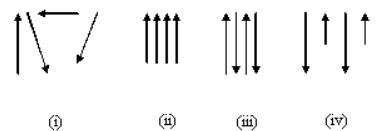## Classify the magnetic materials, Electrical Engineering

Assignment Help:

Classify the magnetic materials into diamagnetic, paramagnetic, ferromagnetic and ferrimagnetic materials. Also give examples of each.

Classification of magnetic materials:-

Magnetic materials for that linear relationship in between M and H exists are divided in classes depending on the sign of χ (magnetic susceptibility). Materials that have negative value of χ the order of 10-4 to 10-6 are termed as diamagnetic and those that have a positive value of χ of about similar order of magnitude are termed as paramagnetic. When the relationship in between M and H is non-linear and shows hysterises effect, this group of materials wherein the resultant magnetization is one to some orders of magnitude. Such materials are called Ferromagnetic materials.

The other classification of magnetic materials contains in the presence or absences of permanent magnetic dipolar in them, Materials, that lack permanent magnetic dipoles, are termed as diamagnetic. When permanent magnetic dipoles are present in the atoms of a material, this may be ferromagnetic, paramagnetic, anti- ferromagnetic of ferrimagnetic depending upon the interaction among the individual dipoles. Therefore, if the interact between the atomic permanent dipoles moments is negligible or zero and the individual dipole moments are oriented at random as demonstrated in figure (i).That material will be paramagnetic. If the dipole interacts in which a manner which they tend to line up in parallel, as demonstrated in figure (ii), the material will be ferromagnetic. If neighbouring moments are aligned anti parallel as demonstrated in figure (iii), the materials are anti- ferromagnetic. There is a large resultant magnetization, in ferromagnetic materials whereas in anti- ferromagnetic materials the magnetisation vanishes. While the order of the magnetic moments is as demonstrated in figure (iv), the phenomena is termed as ferrimagnetisms.

Illustrations of diamagnetic materials are Diamonds, copper and Graphite. Illustrations of paramagnetic materials are Ebonite and platinum and also Aluminium. Illustrations of Ferromagnetic materials are cobalt, Nickel and Iron.

#### Determine and sketch il for inductance, Q. For the circuit of Figure, deter...

Q. For the circuit of Figure, determine and sketch i L (t) and vC(t) for inductance values of (a)3/4 H, (b) 2/3 H, and (c) 3/17 H. Note that the inductance values are chosen her

#### Zener Diode, A Zener diode has the specifications VZ=6.8V and PDmax.=500mW....

A Zener diode has the specifications VZ=6.8V and PDmax.=500mW. Assume RZ=0. (a) Find the maximum allowable current iZ when the Zener diode is acting as a regulator. (b) If a sing

#### Develop a general expression for the force, Consider an electromagnet, as s...

Consider an electromagnet, as shown in Figure, which is used to support a solid piece of steel and is excited by a coil of N = 1000 turns carrying a current i = 1.5 A. The cross-se

ohm''s law

#### Determine the current in the circuit and the voltage, Q. Two identical junc...

Q. Two identical junction diodes whose volt-ampere relation is given by Equation in which I S = 0.1 µA, V T = 25 mV, and η = 2, are connected as shown in Figure. Determine the cu

#### Obtain rated fundamental voltage across the motor, A 440-V, 60-Hz, six-pole...

A 440-V, 60-Hz, six-pole, wye-connected, squirrel-cage induction motor with a full-load speed of 1170 r/min has the following parameters per phase referred to the stator: R 1 = 0.

#### How can magnetic fields can be plot, The magnetic fields can be plot by usi...

The magnetic fields can be plot by using: i.  Compass ii.  Iron dust method  .

#### What is transient program area, What is TPA (transient program area)? T...

What is TPA (transient program area)? The memory system is divided in three major parts: transient program area, System is and XMS that is extended memory system.

#### What do you mean by insurance, Q. What do you mean by Insurance? This i...

Q. What do you mean by Insurance? This is done by creating a common fund out of the contribution (known as premium) from several persons who are equally exposed to the same los

#### Show demagnetizing effect of armature reaction, Q. A20-hp, 250-Vshuntmotor ...

Q. A20-hp, 250-Vshuntmotor has a total armature - circuit resistance of 0.25  and a field-circuit resistance of 200.At no load and rated voltage, the speed is 1200 r/min, and the